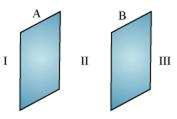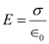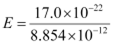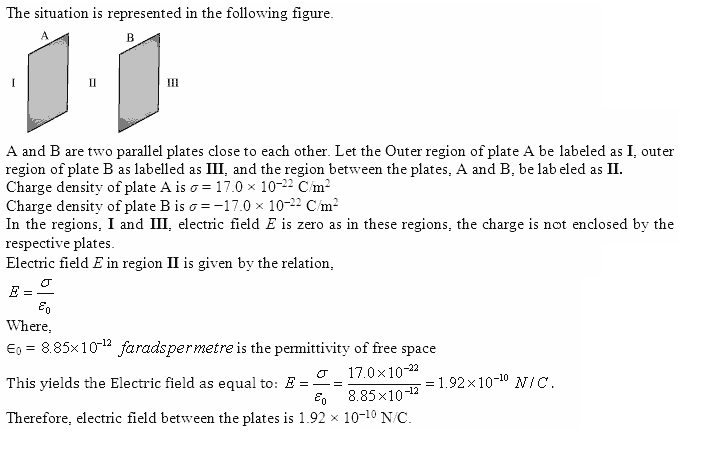## Thursday, 6 June 2013

### Two large, thin metal plates are parallel and close to each other. On their inner faces, the plates have surface charge densities of opposite signs and of magnitude 17.0 × 10−22 C/m2. What is E: (a) in the outer region of the first plate, (b) in the outer region of the second plate, and (c) between the plates?

Two large, thin metal plates are parallel and close to each other. On their inner faces, the plates have surface charge densities of opposite signs and of magnitude 17.0 × 10−22 C/m2. What is E: (a) in the outer region of the first plate, (b) in the outer region of the second plate, and (c) between the plates?

Method - 1

The situation is represented in the following figure.A and B are two parallel plates close to each other. Outer region of plate A is labelled as I, outer region of plate B is labelled as III, and the region between the plates, A and B, is labelled as II.

Charge density of plate A, σ = 17.0 × 10−22 C/m2 Charge density of plate B, σ = −17.0 × 10−22 C/m2

In the regions, I and III, electric field E is zero. This is because charge is not enclosed by the respective plates.

Electric field E in region II is given by the relation,Where,

0 = Permittivity of free space = 8.854 × 10−12 N−1C2 m−2= 1.92 × 10−10 N/C

Therefore, electric field between the plates is 1.92 × 10−10 N/C.
Method - 21.2.